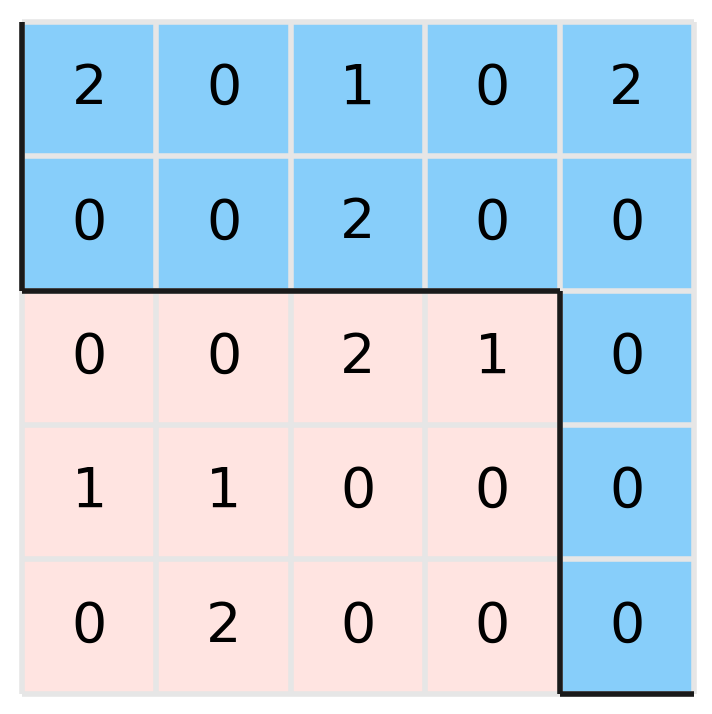M - Even split

Languages: C, C++, Java, Python, ... (details)

You are given a matrix $A$ where each cell has a value of $0$, $1$ or $2$. We want to divide the matrix into two parts, from the vertex at position $(0,0)$ to the vertex at $(n, m)$ by a sequence of edges, in such a way that edge $a$ precedes edge $b$, if $b$ is to the right or below of $a$ and they share the vertex on the right or below of edge $a$. Is it possible to do the division in such a way that on both sides the sum of the values of the cells are the same?Example test case 3

Input

First line contains 2 integers $n$ y $m$ ($1 \leq n, m \leq 1000$),  the amount of rows and columns of the matrix respectively.

Then $n$ lines, each one with $m$ integers $A_{i,j}$ ($0 \leq A_{i,j} \leq 2$), indicating the value of the cell $j$-th on the row $i$-th.

Output

Print 'YES' if it is possible to find a path that satisfies the conditions described above; otherwise print 'NO'. If the answer is 'YES', print a second line with a string of length $n+m$ consisting of characters R' (right) or D' (down) that describes a possible path. If there are multiple paths satisfying the above conditions, print any of them.

Sample test(s)

Input
2 2 2 2 1 1
Output
YES RDDR
Input
3 3 0 1 1 2 0 0 2 0 0
Output
NO
Input
5 5 2 0 1 0 2 0 0 2 0 0 0 0 2 1 0 1 1 0 0 0 0 2 0 0 0
Output
YES DDRRRRDDDR
Input
2 3 0 0 0 0 0 0
Output
YES DDRRR